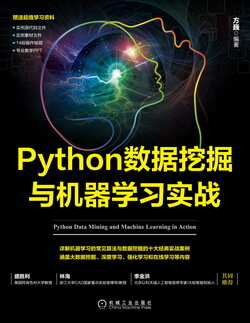# Python 数据挖掘与机器学习实战 (35)：Python 语言简介 2.6(Python 的字典)

dict = {key1 : value1, key2 : value2 }

dict = {'a': 1, 'b': 2, 'b': '3'}print(dict['b'])print(dict)

3{'a': 1, 'b': '3'}

dict = {'Alice': '20', 'Beth': '21', 'Cecil': '22'}

dict1 = { 'abc':123}dict2 = { 'abc': 123, 98: 37 }

dict = {'Name': 'xioaming', 'Age': 20, 'Class': 'First'}print ("dict['Name']: ", dict['Name'])print ("dict['Age']: ", dict['Age'])

dict['Name']:  xioamingdict['Age']:  20

dict = {'Name': 'xioaming', 'Age': 20, 'Class': 'First'}print ("dict[xiaowang']: ", dict['xiaowang'])

Traceback (most recent call last):  File "F:/program/2.6.py", line 19, in <module>    print ("dict[xiaowang']: ", dict['xiaowang'])KeyError: 'xiaowang'

dict = {'Name': 'xioaming', 'Age': 20, 'Class': 'First'}dict['Age'] = 22                        #修改年龄dict['School'] = "NUIST"                 #添加新的键 / 值对print ("dict['Age']: ", dict['Age'])print ("dict['School']: ", dict['School'])

dict['Age']:  22dict['School']:  NUIST

dict = {'Name': 'xioaming', 'Age': 20, 'Class': 'First'}del dict['Name']                     #删除键是'Name'的条目dict.clear()                         #清空词典所有条目del dict                            #删除词典print ("dict['Age']: ", dict['Age'])print ("dict['School']: ", dict['School'])

Traceback (most recent call last):  File "F:/program/DCGAN-tensorflow-master/2.6.py", line 34, in <module>    print ("dict['Age']: ", dict['Age'])TypeError: 'type' object is not subscriptable